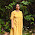## Thursday, 28 May 2015

In a couple of earlier posts, we have seen how Google Charts can be used to generate online charts and graphs but the challenge was that were was a lot of rather messy javascript to play around with. All such mess has been rendered redundant with the R package googleVis and it is now possible to generate charts with the Google Charts API using only R.

The resultant charts need to be viewed in a browser -- and the html code is available for embedding in websites like this. This google docs spreadsheet has some data on milk production in Indian states. It has been downloaded and used for these exercises

Here is the code :

``````
usePackage <- function(p) {
if (!is.element(p, installed.packages()[,1]))
install.packages(p, dep = TRUE)
require(p, character.only = TRUE)
}

StateMilkTotal <- StateMilk[, c("State","Total")]
StateName <- StateMilk[, "State"]

GeoStates <- gvisGeoChart(StateMilk, "State", "Total", options=list(region="IN", displayMode="markers", resolution="provinces", width=1200, height=800))
plot(GeoStates)
GeoStates2 <- gvisGeoChart(StateMilk, "State", "Total", options=list(region="IN", displayMode="regions", resolution="provinces", width=600, height=400))
plot(GeoStates2)
print(GeoStates2)
```
```

and here is the chart that is generated by code printed out by the last print command
-----------------
Data: StateMilk • Chart ID: GeoChartIDe315e03b5c4googleVis-0.5.8
-----------------

In addition to maps, you can also draw bar and column charts like with this code
``````
MilkBar <- gvisBarChart(StateMilk, xvar="State", yvar=c("CowMilkTotal", "BuffaloMilk"),options = list(width=800, height=900))
plot(MilkBar)

MilkColumn <- gvisColumnChart(StateMilk, xvar="State", yvar=c("CowMilkTotal", "BuffaloMilk"), options = list(width=800, height=900) )
plot(MilkColumn)
```
```

A complete list of sample code for all possible types of googleVis charts is available at on this page. All the examples can be executed by running the demo program as explained at the top of the reference page.

1.2.